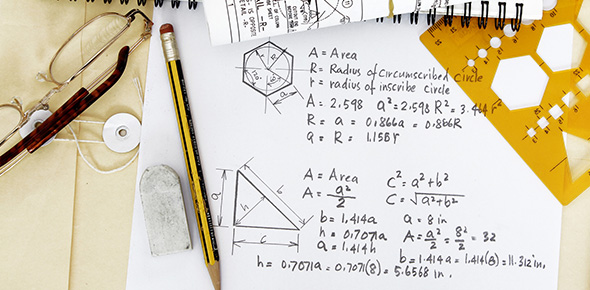4 QuestionsSettingsThis is a quiz to assess students' abilities to calculate the area of various geometric two-dimensional shapes.

Related Topics
• 1.
What is the area of a rectangle with side lengths 6 cm and 8 cm?
• A.

48 cm squared

• B.

48 cm

• C.

14 cm

• D.

28 cm squared

• 2.
What is the formula for calculating the area of a rectangle?
• 3.
What is the area of a square with a side length of 5 inches?
• A.

25 inches squared

• B.

20 inches squared

• C.

20 inches

• D.

25 inches

• 4.
What is the formula used to calculate the area of a parallelogram?